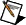# Integral Gain (Ki)

Determines the contribution of restoring force that increases with time and thus ensures that the static position error in the servo loop is forced to zero. This restoring force works against constant torque loads to help achieve zero position error when the axis is stopped.

Each sample period, the position error is added to the accumulation of previous position errors to form an integration sum. This integration sum is scaled by dividing by 256 prior to being multiplied by Ki. Therefore, the formula for calculating the integral contribution in the 16-bit DAC command output is as follows:where LIMIT = shorthand for the effects of the integration limit described in the following sections.Note  The scaling by 1/256 allows the use of integer values for the integral gain even when only a small amount of integral contribution is required.

In applications with small static torque loads, this value can be left at its default value of zero (0). For systems having high static torque loads, this value must be tuned to minimize position error when the axis is stopped.

Non-zero values of Ki, while reducing static position error, tend to increase position error while accelerating and decelerating. This effect can be mitigated through the use of the Integration Limit parameter. Too high a value of Ki often results in servo loop instability. For these reasons, it is recommended that Ki be left at its default value of zero until the servo system operation is stable and then you can add a small amount of Ki to minimize static position errors.Note  Ki has no effect when Ilim is equal to zero.